## Introduction to Rotationally Symmetric Irregularity

Category: Product NewsRotationally Symmetric Irregularity (RSI) is a defect that can accumulate in optical systems with many components and degrade the performance of the system.  In this blog post, we introduce RSI and how it is specified per ISO 10110.  We discuss how to model RSI using the Zernike Standard Sag surface in OpticStudio.

Rotationally Symmetric Irregularity (RSI) refers to a group of rotationally symmetric errors in the shape of an optical surface. The errors are represented by Zernike polynomials and have the form of third-order spherical aberration and higher orders of spherical aberration.

RSI polynomial forms

The polynomial forms referred to as RSI are: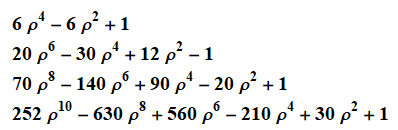In terms of Zernike Standard Coefficients as defined in OpticStudio, the Zernike polynomials involved in RSI are:Why RSI is a concern

RSI terms can quickly become problematic in any system with a large number of surfaces, such as endoscopes, projection lenses, or photolithography lenses.

It is common to assume that the surface errors in such systems combine randomly, and so an estimate of the total error is a Root Sum Square (RSS) of each error type.  This is not true for the RSI polynomials. The surface errors don’t add directly, but the total error is larger than an RSS calculation suggests. For example, the four RSI polynomial terms are shown below, each with a coefficient value of 0.01.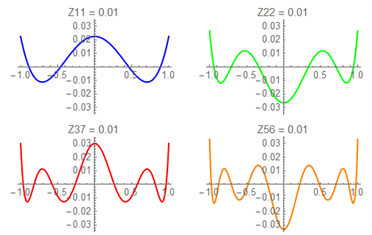The sum of the above four polynomials is shown below. The RSS of the polynomials is 0.1, but the true RSS surface error is 0.14.(Notice, for the graphics above, that the RSI polynomials can be used to capture edge roll on a surface. Edge roll is a common manufacturing difficulty.)

Specifying RSI on a drawing per ISO 10110

The definition of the RSI terms is given in ISO 10110 Part 5:  Surface form tolerances.  The most basic form of RSI used on optical drawings is:where the terms are defined as:    The P-V power error on the surface, infringes or nanometers
B    The total P-V surface irregularity, infringes or nanometers
C    The P-V RSI, infringes or nanometers

There are many variants on this basic form, as specified in ISO10110-5. A test wavelength may be specified. A limit on the total RMS of the surface error can also be included.

The specification:Indicates a P-V power error of 3 fringes, a total P-V irregularity less than 1 fringe, and the P-V RSI at less than 0.5 fringes. The P-V values are measured at a wavelength of 500 nm.

These limits apply to the optical surface itself. A fringe is defined as extending from dark center to dark center in an interferogram.  One fringe corresponds to wavelength/2.

It is frequently clearer to specify the surface error in nanometers. The equivalent specification in nanometers is: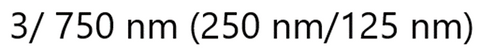Zernike polynomials in ISO 14999-4

The Zernike polynomials are defined in ISO 14999 Part 4:  Interpretation and evaluation of tolerances specified in ISO 10110
The polynomials are referred to using their (n,m) indices:  Z(0,0), Z(1,1), Z(1,-1), etc.#### Modeling RSI in OpticStudio

In OpticStudio, the Zernike Standard Sag surface can be used to represent a surface that contains RSI and other surface errors.  The Zernike Standard Sag surface can accommodate a base shape that is a conic or an even asphere, and Zernike terms are added to the base shape.

For surfaces more complex than a conic or an even asphere, the Zernike Standard Phase surface can be used to add errors to the surface.

A Zernike Standard Sag surface is shown below, in the Lens Data Editor (LDE) of OpticStudio. The Zernike coefficients appear at the far right of the LDE.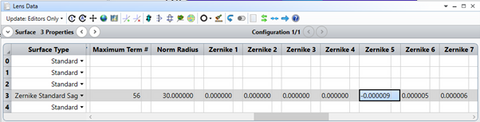The definition of the Zernike Standard Sag polynomials is given in the OpticStudio Help files.  Part of the list is shown below.  Because the terms are slightly different than the definitions used in ISO 14999-4, translation is required.  For example, the ISO 10110 term, Z(4,0), is represented here by ZOS Standard Zernike term 11.The conversion from ISO 10110 Zernike terms for RSI to the Zernike Standard Sag surface is summarized below.  Zernike’s 11, 22, 37, and 56 are needed.  The parameter and column numbers in the LDE are also listed since those quantities are useful for programmatically controlling the RSI.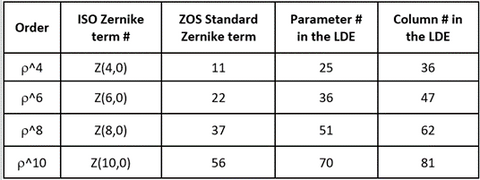#### Modeling RSI

We can model a surface that contains the maximum allowed amounts of RSI and irregularity using the following steps:

• Assign random values to the RSI terms: Z11, Z22, Z37, and Z56.
• Check the RMS surface error.
• Scale to achieve the correct value for C.
• This will take just a single scaling because scaling each Zernike coefficient by a constant scales the RMS by the same constant.
• Assign random values to other Zernike polynomials to model B, total irregularity.
• Without disturbing the values of the RSI terms: Z11, Z22, Z37, and Z56.
• Scale the Zernike terms to reach the correct total irregularity.
• This will be an iterative process since the values of the RSI terms can’t be changed.

This process is best carried out programmatically. The Zemax Programming Language (ZPL) can be used. Or the Application Programming Interface (API) in OpticStudio can be used.  An example of modeling the irregularity and RSI for a parabolic mirror using the API will be in next week’s blog post!

For tolerancing total surface irregularity and RSI (B and C), random values can be selected for B and C. And the above process is carried out again but using the randomly generated values of B and C rather than the maximum allowed values.